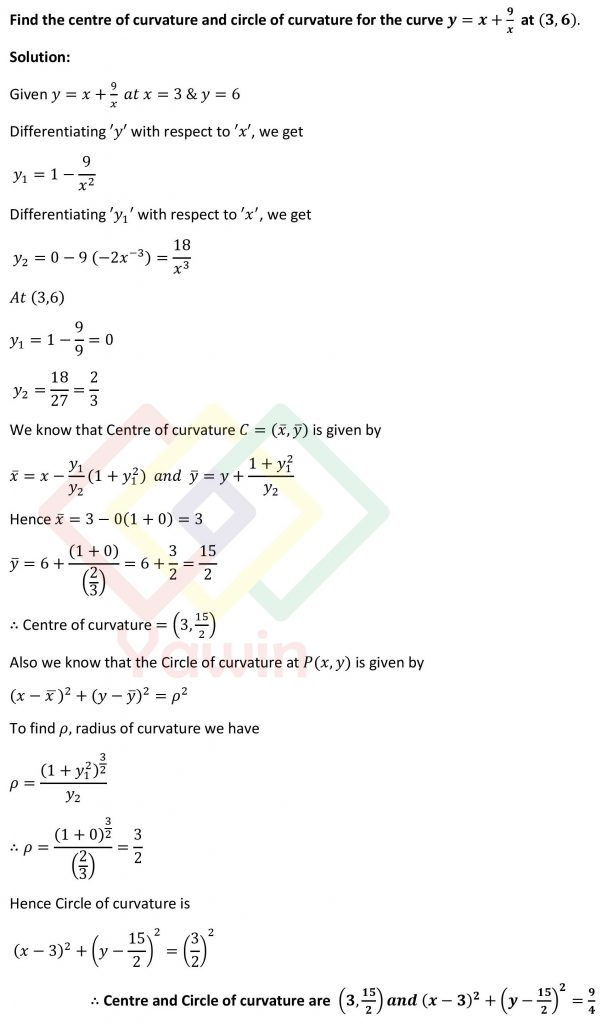Find the centre of curvature and circle of curvature for the curve y=x+9/x at (3,6)

## Question## Question

Find the centre of curvature and circle of curvature for the curve y=x+9/x at (3,6)

## Topic

Radius of curvature, Centre of curvature, Circle of curvature